# Key Types of Regressions: Which One to Use?So, regression… aside from other algorithms and statistical models, it is one more building block upon which Machine Learning successfully works. In its core, regression aims to find the relationship between variables and for Machine Learning it is needed for predicting the outcome based on such a relationship.

Obviously, any self-respecting ML-engineer has to be well-oriented in this subject. But wait, there is a whole slew of regressions. Linear and Logistic regressions are ordinarily the first algorithms people learn. But, the truth is that some innumerable forms of regressions can be performed. Each form has its own importance and a specific condition where they are best suited to apply. So, which one to use?

In this article, I have explained the most commonly used forms of regression in an understandable way, so you can calculate what is most suitable for your specific task.

Let’s roll.

# 1. Linear regression

Equally known as ordinary least squares (OLS) and linear least squares, — the “most classical” type, which appeared nearly 250 years ago (can you imagine?). You can employ it to carry out calculations on small data sets even manually. Current use cases include interpolation, but linear regression is unsuitable for real-world forecasts and proactive analysis.

Plus, in working with modern data characterized by a very chaotic structure, this type of regression is prone to “lag”: linear regression over-accuracy, when a model works too well on one set of data, and very badly on another, while it should describe general patterns, makes it unstable in almost all cases.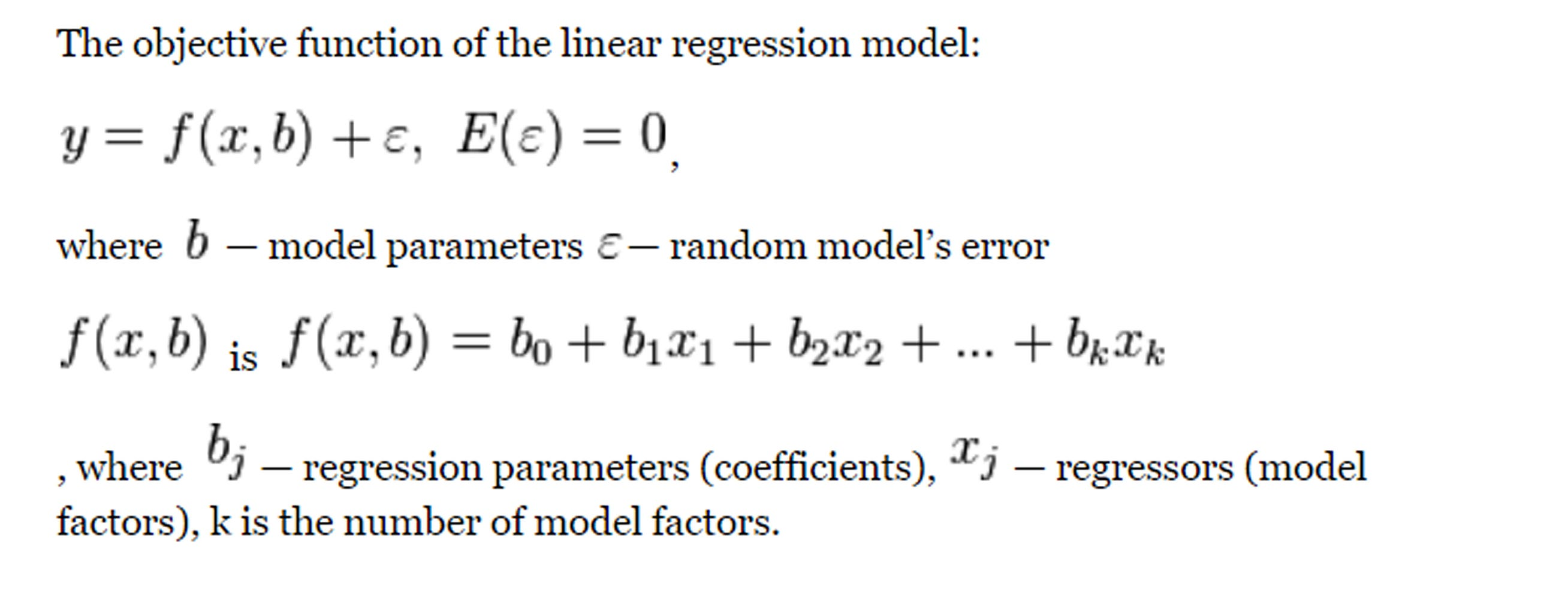# 2. Ridge regression

It is an improvement to linear regression with increased error tolerance, which imposes restrictions on the regression coefficients in order to get a much more realistic result. In addition, this result is much easier to interpret. The method is used to combat data redundancy when independent variables correlate with each other (multicollinearity).

Ridge regression involves estimating parameters using the following formula: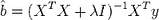# 3. Lasso-regression

It is similar to ridge, except that the regression coefficients can be zero (some of the signs are excluded from the model).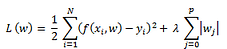# 4. Partial least squares (PLS)

It is a regression is useful when you have very few observations compared to the number of independent variables or when your independent variables are highly correlated. PLS decreases the independent variables down to a smaller number of uncorrelated components, similar to Principal Components Analysis. Then, the procedure performs linear regression on these components rather the original data. PLS emphasizes developing predictive models and is not used for screening variables. Unlike OLS, you can include multiple continuous dependent variables. PLS uses the correlation structure to identify smaller effects and model multivariate patterns in the dependent variables.

# 5. Logistic regression

It is widely used in clinical trials, quantification, and, for example, fraud when the answer can be obtained in binary form (yes/no) for a test drug or credit card transaction. It has some drawbacks inherent in linear regression — low error tolerance, dependence on the data set, but in general, it works better and can be reduced to a linear regression type to simplify the calculations. Some versions — for example, Poisson regression — are improved for use in case of need to get a non-binary answer — classification, age groups, and even regression trees.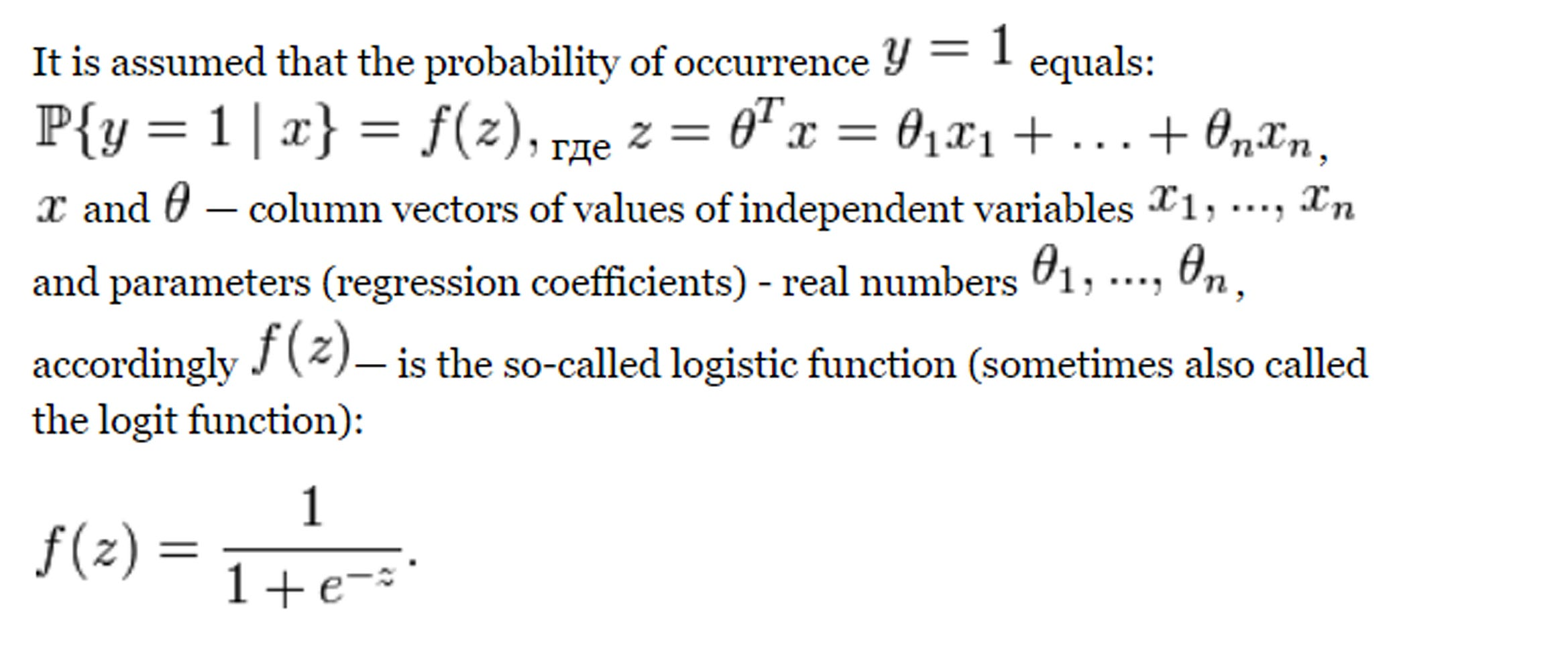# 6. Ecological Regression

It is used in cases where the data is divided into fairly large layers or groups (the regression is applied to each of them separately) — for example, this type of regression is used in political science to assess the group behavior of voters based on summary data. However, one should beware of the “curse of big data”: if millions of regressions are counted, some of the models may be completely inaccurate, and successful models will be “crushed” by noisy models with a high (and naturally artificial) degree of agreement. Therefore, this type of regression is not suitable for predicting extreme events (earthquakes) and studying causal relationships (global warming).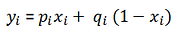# 7. Bayesian linear regression

It is similar to the ridge regression, but it is based on the assumption that all the possible errors will have a normal distribution. Accordingly, it is assumed that a general understanding of the data structure already exists, and this makes it possible to obtain a more accurate model (especially in comparison with linear regression).

However, in practice, if we are dealing with big data, the initial knowledge about the data cannot boast of accuracy, so the assumption is based on conjugate values, that is, it is artificial in its essence — and this is a significant drawback of this type of regression.

The observed variable is calculated as: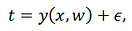the error is distributed normally: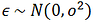# 8. Quantile regression

It is used in connection with extreme events — this type involves the deliberate introduction of bias in the result, increasing the accuracy of the model.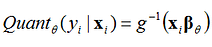# 9. Least absolute deviations (LAD)

Also known as least absolute errors (LAE), least absolute value (LAV), least absolute residual (LAR), sum of absolute deviations, or the L1 norm condition, it is the smallest modulus method) is used to estimate unknown values from measurements containing random errors, as well as to approximate the representation of a given function more simple (approximation). It looks like linear regression but uses absolute values instead of squares — as a result, the model’s accuracy increases without complicating the calculations.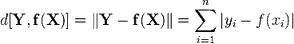# 10. Jackknife resampling (a compact folding knife)

It is a new type of regression used for clustering and data thinning. At the same time, the folding knife does not have the drawbacks of the classical types, providing an approximate, but a very accurate and error-resistant solution to regression problems works well with “independent” variables that correlate or cannot “boast” the normal distribution. This type of regression is considered ideal for black-box type prediction algorithms — it perfectly approximates linear regression without loss of accuracy and works even in cases where traditional regression assumptions (non-correlating variables, normal distribution of data, constant conditional variance) cannot be accepted due to the nature of the data.

Suppose the sample is as follows: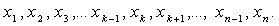In the probabilistic-statistical theory, we assume that this is a set of independent identically distributed random variables. Suppose we are interested in these statistics: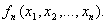The idea that John Tukey proposed in 1949 (this is the “folding knife method”) is to do a lot from one sample, excluding one observation (and returning those that were previously excluded). We list the samples that are obtained from the original: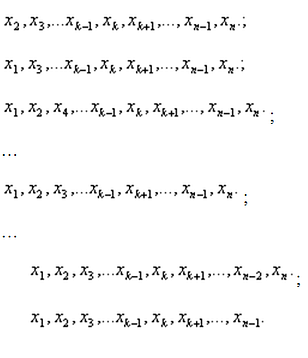Total n new (multiplied) samples of size (n-1) each. For each of them, you can calculate the value of statistics of interest to the econometrics (with a sample size reduced by 1):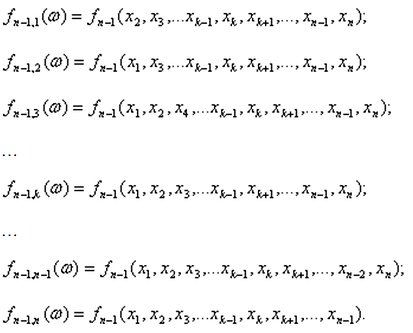The obtained values of the statistics allow judging about its distribution and about the characteristics of the distribution — about the expectation, median, quantile, scatter, mean square deviation.

# Conclusion: What type of regression to choose?• In case of models that require a continuous dependent variable:

Linear regression is the most common and most straightforward to use. If you have a continuous dependent variable, linear regression is probably the first type you should consider. However, you should pay attention to several weaknesses of Linear regression like sensitivity to both outliers and multicollinearity. In this case, it is better to use more advanced variants of Linear regression like Ridge regression, Lasso-regression and Partial least squares (PLS).
• In case of models that require categorical dependent variables:

Pay attention to Logistic Regression. This model is the most popular for binary dependent variables. It is highly recommended to start from this model setting before more sophisticated categorical modeling is carried out. A categorical variable has values that you can put into a countable number of distinct groups based on a characteristic. Logistic regression transforms the dependent variable and then uses Maximum Likelihood Estimation, rather than least squares, to estimate the parameters.
• In case of models that require a count dependent variables:

Use Poisson regression. Count data frequently follow the Poisson distribution, which makes Poisson Regression a good possibility. With a Poisson variable, you can calculate and assess a rate of occurrence.

…………………………………

Do I miss anything? Disagree entirely? Share your opinion in the comments! Feel free to follow me on
Medium and Instagram.

“Key Types of Regressions: Which One to Use?”
– Oleksii Kharkovyna

1.ds1
over 1 year ago
Linear regression :)

### Related Stories

Nov 25, 2021

#### 5 Tips To Ace Your Job Interview For A Data Scientist Opening

5 Tips To Ace Your Job Interview For A Data Scientist Opening.PNG 795.94 KBImage SourceAspiring data scientists have a bright future ahead of them....By Daniel Morales
Nov 12, 2021

#### When to Avoid Deep LearningBy Matt Przybyla
Oct 16, 2021

#### 6 Advanced Statistical Concepts in Data Science

The article contains some of the most commonly used advanced statistical concepts along with their Python implementation.In my previous articles Be...By Nagesh Singh Chauhan